RocFall3 | Your new tool for 3D assessment of slopes at risk for rockfalls. Get started with RocFall3 today!

# Axisymmetric Analysis

What boundary conditions should be used?

For an axisymmetric model, when the left boundary is at x =0, the left edge should be fixed in the x direction, and free to move in the y-direction. The left boundary can be free in both directions when it is not at x=0.

The bottom is usually fixed in the y direction. The top will be free if you are excavating down from the ground surface and fixed in the y direction if you are starting from some deeper location.

The boundary we get most questions about is the right edge. Should it be fixed in the x and y direction, or only in x? The answer is that neither approach is perfectly accurate.

You are trying to simulate a boundary that is an infinite distance away from the shaft. If we fix the right boundary in x and y, the condition is too rigid. If we fix only in x, then the boundary is 'too free'. The real solution will be bracketed by these two approaches.

Note that the further the boundary is from the shaft, the less important this boundary condition is.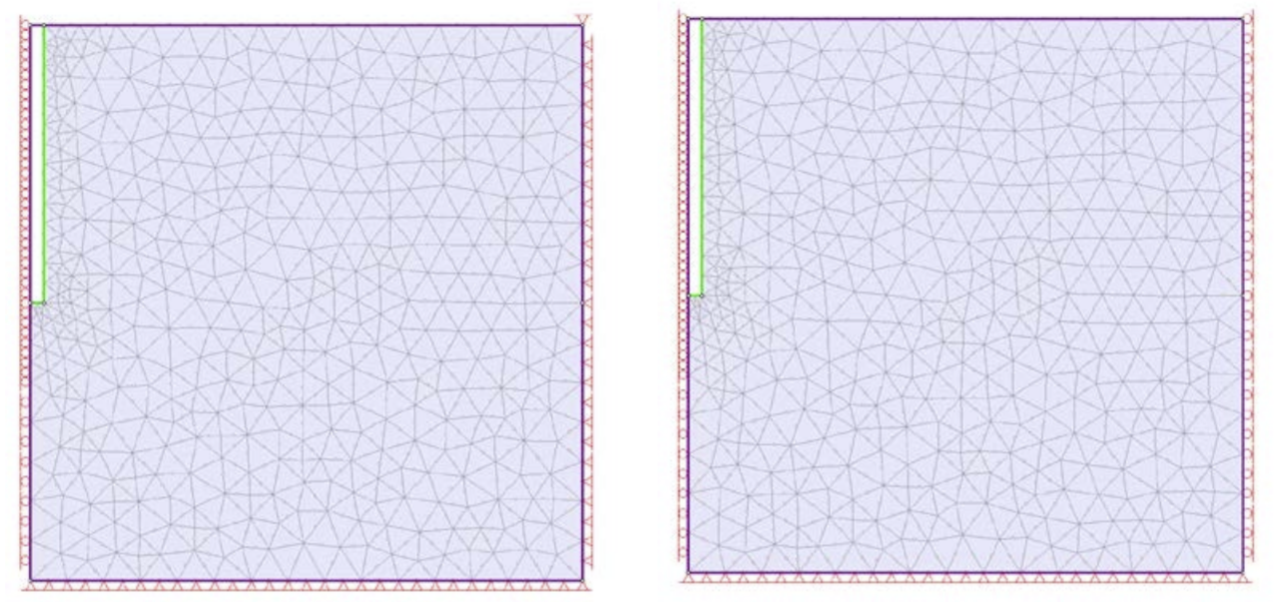Two possible approaches for modelling a shaft excavated down from the ground surface

Why is my mesh not graded?

In an axisymmetric analysis, there is no excavation boundary (i.e. an excavation boundary according to the boundary types defined in RS2). The excavation is open at the left edge of the model so the excavation must be implicitly defined by the external boundary, and material or stage boundaries. When RS2 generates a graded mesh, it grades from an excavation boundary. With no excavation boundary, there is no grading.

You will therefore want to manually increase the density of the mesh around your shaft in one of the following ways:

• Specify discretization regions under the advanced option in the mesh dialog
• Use custom discretization
• Use the 'Increase Discretization Density' and 'Increase Mesh Element Density' options under the Mesh menu

Ungraded mesh (left) and mesh with increased discretization density around the shaft (right).

I want to apply liner support to my shaft. What should I use for Area and Moment of Inertia?

You should enter the same area and moment of inertia that you would for a plane strain analysis.

How do I interpret the resulting liner forces?

In a plane strain analysis, the liner forces are given per unit length in the third dimension. In an axisymmetric analysis, the liner forces are per unit arc length of rotation. This has the following consequence for your interpretation. Imagine that your liner represents a line of piles and you want to know the axial force on each pile. For a pile spacing , the number of piles in one distance unit is: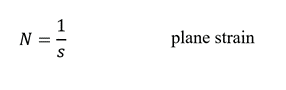In the axisymmetric case, the number of piles in one arc length unit is: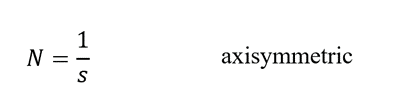In both cases, the force on each pile is simply the total force divided by the number of piles.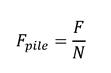so,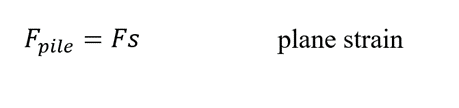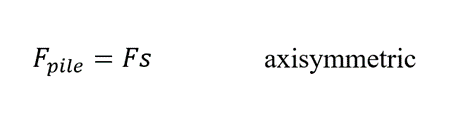where F is the force shown by RS2 for the liner.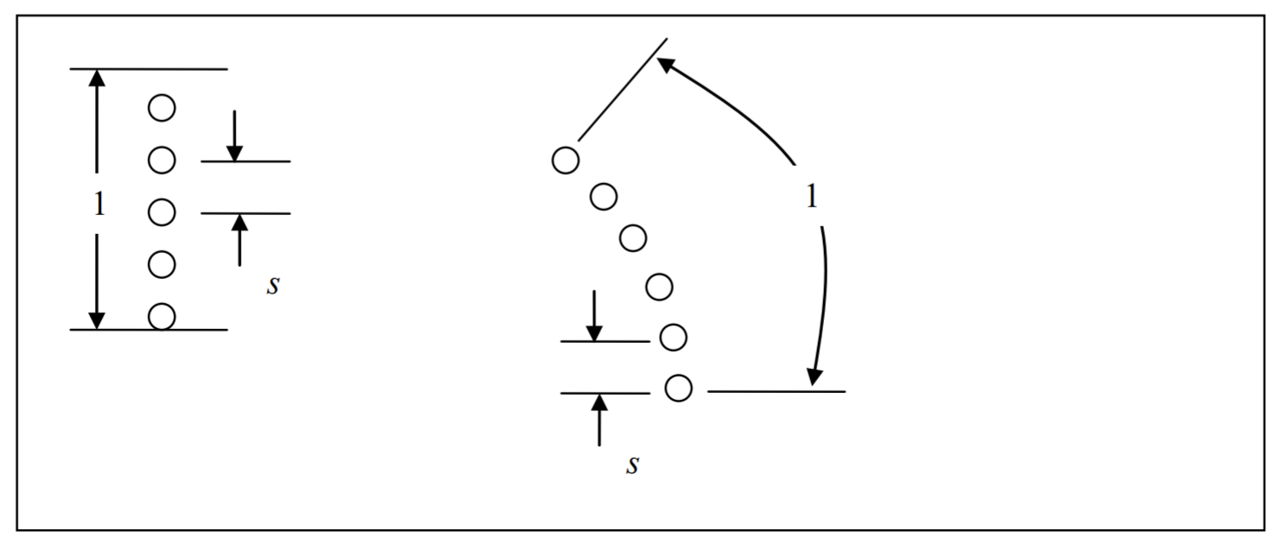A plan view of pile wall in a plane strain analysis (left) and an axisymmetric analysis (right).

What boundary conditions should I use for an axisymmetric seepage analysis?

The right edge should be set to represent the far-field conditions (usually a constant head boundary condition with the head equal to the location of the water table).

For the boundaries of the shaft, the boundary conditions depend on what you want to simulate. If you only want to keep the inside of the shaft dry, you will probably maintain a 0 pressure at the bottom of the shaft through pumping. The water table will intersect the side of the shaft at some unknown location, so set these boundaries to be unknown. The figure below shows an example analysis.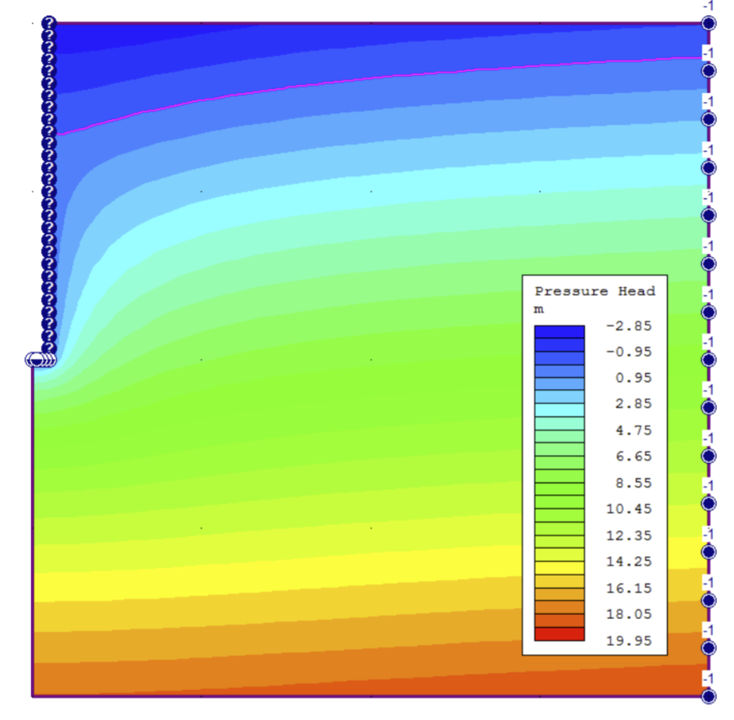If the shaft represents a well being pumped at a known rate, then you probably want to set a normal infiltration rate, , on the well boundary. This is described in verification problems #15 and #16 (here).

How should I interpret the discharge rate?

In an axisymmetric analysis, the discharge rate is given per radian. So for example, the following plot shows a shaft with a discharge section at the bottom. You wish to know the total discharge rate through the bottom of the shaft. Multiply the number shown (5.44e-7 m3 /s) by 2π (radians) to get the total discharge rate: 3.42e-6 m3 /s.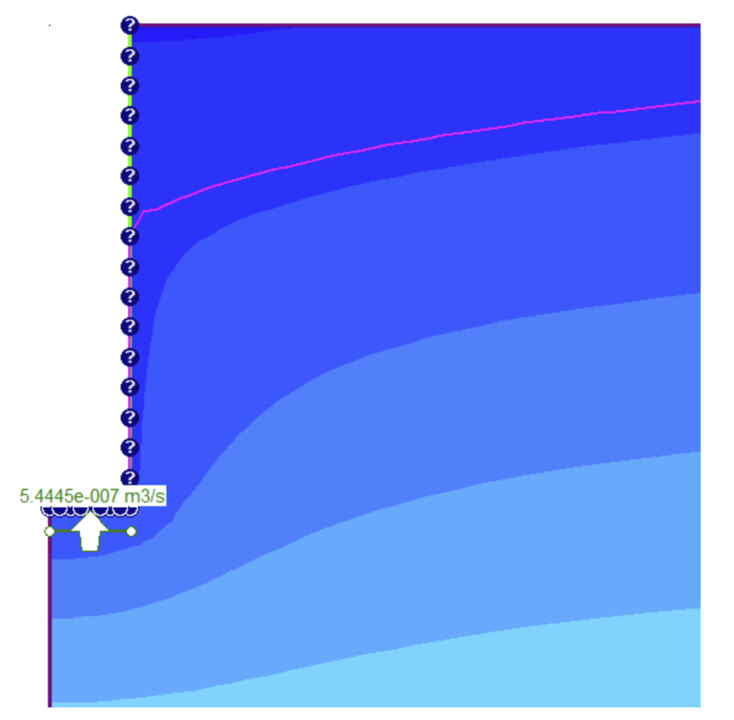The maximum compressive stress (σ1) at the edge of the shaft doesn't seem right.

In RS2, the maximum and minimum compressive stresses (σ1 and σ3) are calculated from the stresses in the observed plane. In an axisymmetric analysis of a vertical shaft, the x-axis of the observed plane is in the radial direction and the y-axis is in the vertical direction. The values calculated for σ1 and σ3 do not consider the circumferential stress acting into the page. If the horizontal stress is high, as in a coal mine for example, the circumferential stress will probably be the maximum stress at the shaft boundary. To observe the circumferential stress, you need to plot σz .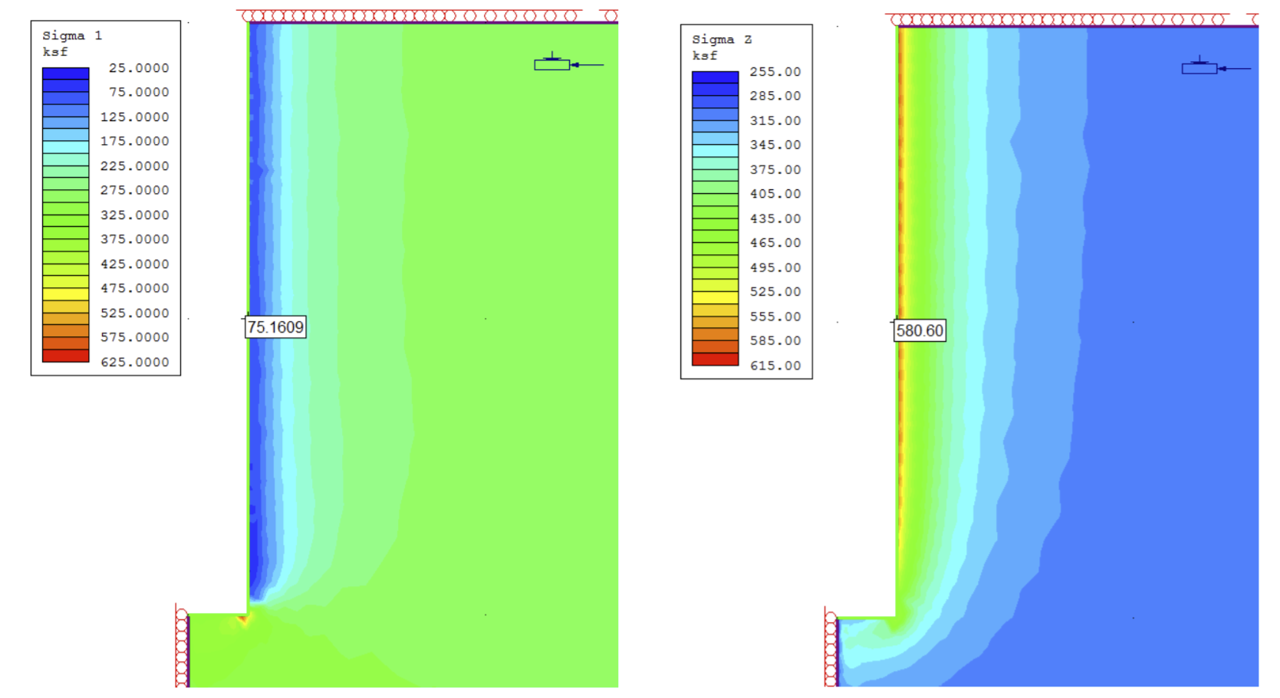σ1 (left) and σz (right) for a shaft in a coal mine. A query point shows the values on the periphery of the shaft.

Can I model a non-circular shaft with axisymmetric analysis?

If the shaft is not circular, you cannot do an axisymmetric analysis. You will have to do a plane strain analysis of a cross section of the shaft. Be aware that the axisymmetric analysis is a three-dimensional analysis that considers the confining effect of the bottom of the shaft. The plane strain analysis does not account for this effect and you will probably want to simulate the excavation of the shaft in the third dimension using techniques described in Tutorial: 3D Tunnel Simulation using Core Replacement.

There are other scenarios which may prevent you from performing an axisymmetric analysis such as:

• The stress field is not aligned with the horizontal and vertical directions
• The stress field is significantly different in the two horizontal directions
• You wish to account for bolts or failing liners

In these cases, you will need to perform a plane strain analysis. However, be aware that when modelling a shaft in plane strain, the true vertical direction is into the page. Since RS2 always assumes gravity acts in the negative y direction (in the 2-dimensional coordinate system):

• The Gravitational field stress option cannot be used. You must use the Constant field stress option, with sigma_z = vertical stress, sigma_1 and sigma_3 = major and minor horizontal principal stresses.
• Vertical groundwater flow (parallel to the shaft) cannot be analyzed. Seepage analysis can be performed in a horizontal plane, with some restrictions. Special attention must be paid to boundary conditions and data interpretation.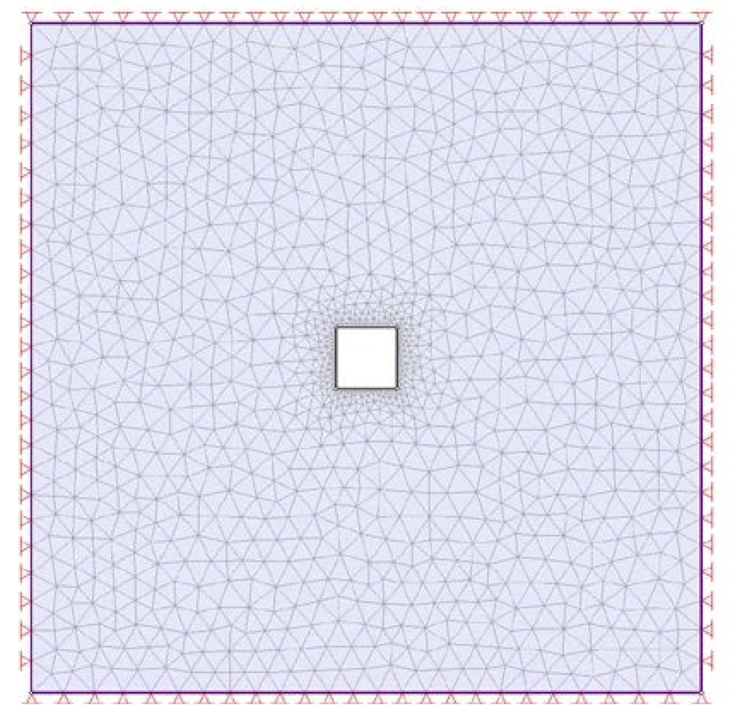Plan view of a shaft with a square cross-section modelled with a plane strain analysis.